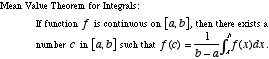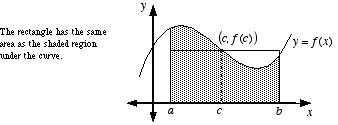# mean value theorem for integrals

Mean Value Theorem for Integrals

A variation of the mean value theorem which guarantees that a continuous function has at least one point where the function equals the average value of the function.